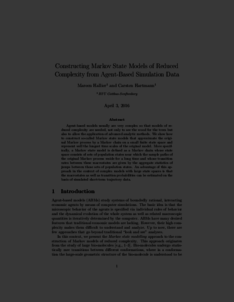Repository: Freie Universität Berlin, Math Department

# Constructing Markov State Models of Reduced Complexity from Agent-Based Simulation Data

Hallier, M. and Hartmann, C. (2016) Constructing Markov State Models of Reduced Complexity from Agent-Based Simulation Data. Social Simulation Conference 2016 . (Submitted)Preview

640kB

## Abstract

Agent-based models usually are very complex so that models of re- duced complexity are needed, not only to see the wood for the trees but also to allow the application of advanced analytic methods. We show how to construct so-called Markov state models that approximate the origi- nal Markov process by a Markov chain on a small finite state space and represent well the longest time scales of the original model. More specif- ically, a Markov state model is defined as a Markov chain whose state space consists of sets of population states near which the sample paths of the original Markov process reside for a long time and whose transition rates between these macrostates are given by the aggregate statistics of jumps between those sets of population states. An advantage of this ap- proach in the context of complex models with large state spaces is that the macrostates as well as transition probabilities can be estimated on the basis of simulated short-term trajectory data.

Item Type: Article Mathematical and Computer Sciences > Operational Research > Operational Research not elsewhere classified Department of Mathematics and Computer Science > Institute of Mathematics > Cellular Mechanics GroupDepartment of Mathematics and Computer Science > Institute of Mathematics > BioComputing Group 1899 Carsten Hartmann 14 Apr 2016 07:20 03 Mar 2017 14:42

Repository Staff Only: item control page